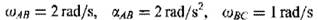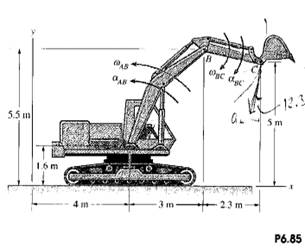### Create an Account

Home / Questions / If the velocity of point C of the excavator in Problem 685 i zero and its acceleration is ...

# If the velocity of point C of the excavator in Problem 685 i zero and its acceleration is Be 4 i mls2 at the instant shown what are WAB WYC and JRC Problem 685 If what is the acceleration

If the velocity of point C of the excavator in Problem 6.85 i zero and its acceleration is Be = 4 i mls2 at the instant shown, what are WAB, WYC and (J.RC?

Problem 6.85

If. what is the acceleration of point C where the .scoop of the excavator is attached?Jun 16 2020 View more View LessSubscribe To Get Solution